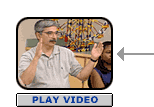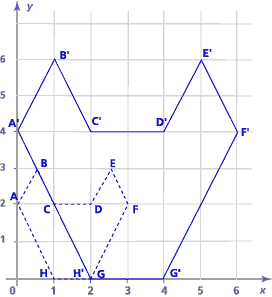# Similarity Part A: Scale Drawings (50 minutes)

## Session 8, Part A

### In this part

• Finding a “Good Copy”
• Doubling the Coordinates
• Distances and Angles

### Finding a “Good Copy”

Here is a picture of a panther:Try to come up with a method of comparing the pictures that will tell you whether a picture is distorted. For example, you may want to look at the ratio of length to height. Looking at angles would be another method.

### Problem A1

Which of these pictures is a good copy of the original picture? Explain how you made your decision.### Video Segment

In this video segment, participants explore different ideas that will help them decide which copy is the best copy of the original picture. Watch this segment after you’ve completed Problem A1.

Compare your method with those the participants used in the video.

You can find this segment in the session video approximately 3 minutes and 3 seconds after the Annenberg Media logo.

Mathematicians use the word “similar” to describe two figures that are the same shape but not necessarily the same size. If one is a scaled copy of the other, then the two figures are similar.

Two polygons are similar if and only if corresponding angles have the same measure and corresponding sides are in proportion.

### Problem A2

Are these two rectangles similar? How do you know?### Problem A3

Are these two triangles similar? How did you decide?Scale Drawings adapted from Connected Geometry, developed by Educational Development Center, Inc. © 2000 Glencoe/McGraw-Hill. Used with permission. www.glencoe.com/sec/math

### Doubling the Coordinates

Here’s a picture of a cat’s head (it’s really a polygon!) on a coordinate grid:### Problem A4

 a. Copy the picture of the cat’s head onto a piece of graph paper, and find the coordinates of each point. b. Multiply the coordinates of each point by 2, creating points A’ through H’. Use the rule (x,y)(2x,2y). c. Plot these new points on the same grid, and connect them in the same order. d. Is your new figure similar to the original picture? How do you know?

### Problem A5

Explain why, if you want to create a similar figure with sides twice as long as the original, you don’t double all the angles as well.

The ratio between corresponding sides of the cat’s head is called the ratio of proportionality. Since we made the sides twice as long, the ratio of proportionality is 2. Note 2

Problem A4 adapted from IMPACT Mathematics, Course 3, developed by Educational Development Center, Inc. © 2000 Glencoe/McGraw-Hill. Used with permission. www.glencoe.com/sec/math

### Distances and Angles

Why does the coordinate trick work to create similar figures?

Rather than looking at the head of a cat, let’s look at a triangle to explain why multiplying the coordinates by 2 creates a similar figure. We need to show two things:

1. The distance between any two points doubles.
2. The angles all stay the same.

Since we know that we can take any polygon and split it up into triangles, this will be enough to show that the scaling trick works for any polygon. If the triangles in the new polygon are all similar to the triangles in the original, then the two polygons themselves must be similar.

Here are two arbitrary points, A = (a1,a2) and B = (b1,b2), on a coordinate grid:### Problem A6

What is the distance between the two points? (Look back to Session 6 if you don’t remember how to find the distance. It might also help to try some specific examples.)

### Problem A7

If you apply the rule (x,y) |→ (2x,2y) to both points to create A’ and B’, what are the new coordinates? What is the distance between A’ and B’? Are you sure that it’s double the distance from A to B?

The reason that the angles don’t change is a little trickier. Here’s the idea: There is some triangle DEF, similar to triangle ABC, but with sides twice as long. (Imagine putting ABC in a copy machine and enlarging it by 100%.) The corresponding angles all have the same measures: m∠A = m∠D, m∠B = m∠E, and m∠C = m∠F.

Because triangles are rigid (SSS congruence), any triangles with the same sides as DEF will also have the same angles as DEF.

In particular, the triangle made by doubling the coordinates of A, B, and C has sides twice as long as triangle ABC. That is, the sides are the same as DEF. So the angles are also the same as DEF and as ABC.

That means the sides are in proportion to the sides of ABC (they’re twice as long) and the angles have the same measures. So the two triangles are similar.

This argument is what we call general in principle. We explained why doubling the coordinates produces similar triangles. But the same argument would work if you multiplied the coordinates by any number.

### Problem A8

How could you modify the definition of similar polygons to test whether figures like the two below are similar?### Note 2

In the cat’s-head problem, you are doing a transformation that’s called dilation. You’ve seen transformations before — such as translation, rotation, and reflection. These “rigid transformations” preserve congruence; when you apply one to a figure, it may move around, but it maintains its size and shape.

Dilation (scaling) can change not just the position of the figure, but also its size. There’s another non-rigid transformation called a shear that can even change the overall shape of a figure — the final product, for example, may have different angle measurements than the original.

### Problem A1

Picture #3 seems to be the most faithful replica of the original picture because proportions between the various body parts are best preserved.

### Problem A2

The two rectangles are similar. Their angles are the same and equal to 90°. To check whether the sides are in proportion, first set up the following ratios of the corresponding sides:If the two are equal, the sides are in proportion. So we have 2/6 = 3/9, and they are both equal to 1/3. So we can say that the corresponding sides are 1:3 ratio.

### Problem A3

The two triangles are similar. By measuring pairs of sides, we can see that they are proportional. In addition, we can measure pairs of corresponding angles and see that they are of equal measure.

### Problem A4

 a. The coordinates of the figure are as follows: A = (0,2) B = (0.5,3) C = (1,2) D = (2,2) E = (2.5,3) F = (3,2) G = (2,0) H = (1,0) b. The coordinates of the new figure are as follows: A’ = (0,4) B’ = (1,6) C’ = (2,4) D’ = (4,4) E’ = (5,6) F’ = (6,4) G’ = (4,0) H’ = (2,0) c. Follow the instructions — see figure below.d. The two figures are similar. We can see this by checking that the corresponding angles have the same measure. The corresponding sides are in a 1:2 proportion by construction.

Problem A5
If you changed the angles, you wouldn’t have the same kind of shape anymore. For example, in a square, all of the angles are 90°. If you double them all, you will simply have a straight line (a bunch of 180˚ angles lined up), which is certainly not “similar” to a square. If we start with a triangle, the angle sum is 180˚. If we double all of the angles, we would end up with something with angles summed to 360° — certainly not a triangle, and not similar to what we started with.

### Problem A6

The distance between the two points is.

### Problem A7

The new coordinates are (2b1,2b2) and (2a1,2a2). The new distance is. The new distance is double the original distance. We can show this by factoring the 22 from the second expression, obtaining the following:.

So the new distance is exactly twice the original distance.

### Problem A8

We would need to find at least two well-defined reference points on the figure (such as the two endpoints of the spirals). In relation to these two points and the line they define, we could establish pairs of corresponding points on the two shapes. We would then require that all of the distances between all pairs of corresponding points be proportional.